## About Nathan Bowler

I'm working at the university of Hamburg on infinite matroid theory.

# A menagerie of infinite matroids

So far in this series we have focused on the search for a good way to axiomatise infinite matroids. Now that we have the axioms, our next aim is to get a better sense of what sort of ground they stake out by taking a whirlwind tour of the known examples of infinite matroids.

Let’s start off with some very familiar examples: The set of subsets of a given set which have size at most $n$,  the set of (edge sets of) forests in a given graph and the set of linearly independent subsets of a given family of vectors in a vector space each give the set of independent sets of a matroid. This is true even if we allow the set, graph or family of vectors in question to be infinite. Of course, all of these examples are finitary (that is, a set is independent as soon as all of its finite subsets are). We saw earlier that it would be a bad idea to only consider finitary matroids, because we would lose the power of duality. But we certainly want our notion of infinite matroid to include all of these examples. Since we now have a concept of infinite matroid which is closed under duality, we get all their duals for free, too.

What about matroids which are neither finitary nor cofinitary? At first it looks like a simple way to construct such examples would be by building infinite uniform matroids. But the usual definition of uniformity, namely that the bases should be all sets of a given size, becomes silly when we replace size’ by cardinality’, since infinite sets can have proper subsets of the same cardinality. It doesn’t help to define things in terms of independent sets. For example, we might hope to have a matroid whose independent sets are just the countable subsets of some uncountable set $E$. But since there are no maximal countable sets, this object wouldn’t even have any bases.

The right notion of uniformity for infinite matroids is as follows: if $B$ is a base and $x$ and $y$ are elements of the ground set $E$ with $x$ in $B$ but $y$ not in $B$ then $B – x + y$ is also a base. It isn’t hard to check that this is equivalent to uniformity when applied to finite matroids. Now we might try to build infinite uniform matroids by just recursively choosing the set of bases, paying attention to the fact that (because of the definition above) whenever we choose a set $B$ we also have to choose all sets $B’$ for which $B \setminus B’$ and $B’ \setminus B$ are finite and of equal size. This simpleminded strategy works, given some weak assumptions [BG15]. Because this recursive construction gives us so much freedom, infinite uniform matroids are much more varied than finite ones.

The flexibility of this construction lets us resolve a very basic question: must it be true that any two bases of an infinite matroid have the same cardinality? Higgs showed that this is true if we assume the continuum hypothesis [H69a]. But using the freedom mentioned above we can also show that it is consistent that the following naive plan for building a matroid with bases of different sizes actually works: run the above recursive construction to build a uniform matroid on an uncountable set, and begin by nominating some countable subset and some uncountable subset as bases [BG15]. So the answer to the question above is actually independent of the usual axioms of set theory.

The other examples we’ll look at today will all be associated to graphs. The finitary matroid mentioned above, whose circuits are (edge sets of) cycles in a given graph $G$, is called the finite-cycle matroid of $G$. There is another finitary matroid whose circuits are the finite bonds of $G$. But these two are no longer dual to each other. The circuits of the dual of the finite-bond matroid correspond to topological circles in a topological space, called the end-compactification of $G$. This space is obtained from $G$ by adding some points at infinity, called ends. These topological cycles’ were actually first invented for a very different reason and helped to motivate the current axiomatisations of infinite matroids. This was the starting point of our story.

So although there is one canonical matroid associated to a finite graph, for an infinite graph we already have two. In fact there are many more. For example, Higgs showed that there very often is a matroid whose circuits are the (edge sets of) finite cycles or double rays in the graph $G$. This doesn’t always work. For example, in the Bean Graph, shown below, the set of bold edges and the set of dashed edges are both bases but there is no way to replace the edge $e$ with a bold edge in the dashed basis and still have a base. So the base axioms don’t hold.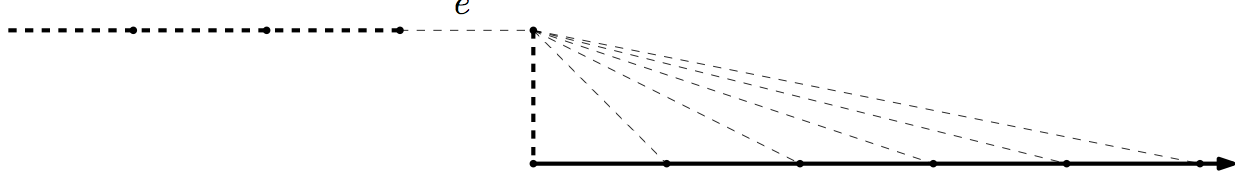This is essentially all that can go wrong: Higgs showed [H69b] that if $G$ includes no subdivision of the Bean Graph then there is a matroid, called the algebraic-cycle matroid of $G$, whose circuits are given as above by the finite cycles and double rays.

Another fertile way to build more matroids from graphs is to ignore some of the ends. More precisely, we consider topological circles in a space obtained from the end-compactification of $G$ by deleting some of the ends. For example, the double ladder has two ends and we might wish to keep only one of them, giving rise to the following space: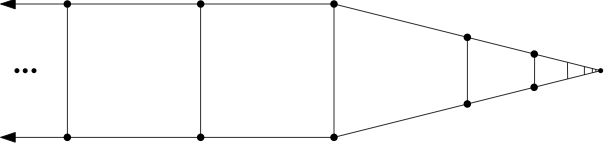Once more it is true that the (edge sets of) topological circles give the circuits of a matroid, as long as the set of ends we remove is Borel [BC15].

Is there any unifying theme to this profusion of examples, except that they can all be built somehow from graphs? Yes! Each of these matroids can represented by a topological space. The finite-cycle matroid of $G$ is represented by the geometric realisation of $G$ and the topological-cycle matroid by the end-compactification. The rich collection of matroids we just discussed are represented by subspaces of the end-compactification. The algebraic-cycle matroid is represented by the space obtained from the end-compactification by identifying all the ends.

More generally, there is a precisely specified class of graph-like topological spaces and a  notion of representation of a matroid by such a space, such that a matroid is representable in this way if and only if, firstly, every intersection of a circuit with a cocircuit is finite (this corresponds to the fact that topological circles are compact), and secondly, it satisfies the usual excluded-minor characterisation of graphic matroids.

Thus the wide range of ways of building infinite matroids from graphs corresponds to the variety of ways of building such graph-like spaces out of graphs. We have seen that this construction doesn’t always give a matroid, as with the Bean Graph above, but it does so often enough to give a rich variety of matroids. For example, we always get a matroid when the space is compact. Hence the (edge sets of) topological circles in the following picture give the circuits of a matroid, the Sierpinski Matroid: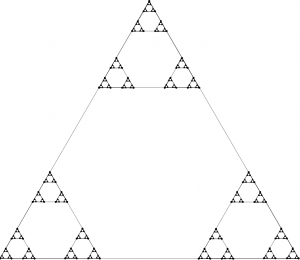Before we conclude, lets just glance at a couple of the other kinds of infinite matroid which have been discovered. There are frame matroids: Matthews and Oxley showed that in any graph $G$ the edge sets of subdivisions of the following graphs gives the set of circuits of a matroid [MO77], and a more general construction works in in infinite biased graphs.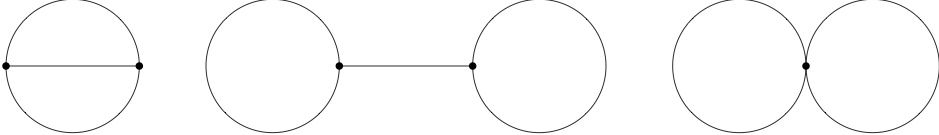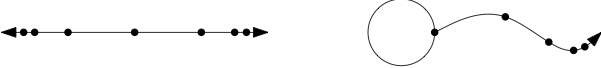There are infinite gammoids, defined either in terms of bipartite graphs or of directed graphs. The question of when these constructions give rise to matroids is a delicate one and has been investigated by Afzali, Law and Müller [ALM15].

Finally, we can define the truncation of a non-free matroid $M$ to have as independent sets the proper subsets of independent sets of $M$, and all truncations and co-truncations of infinite matroids are again infinite matroids. This gives lots of new examples, such as the matroids whose circuits are edge sets of topological copies of the following spaces in a graph-like space: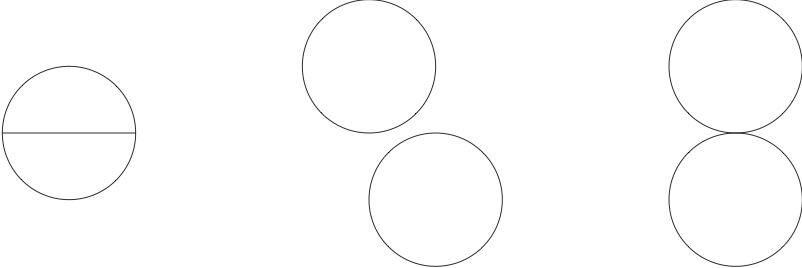This is not so much a class of examples as a way to build new infinite matroids from old. We’ll consider some other fertile ways to combine infinite matroids next time.

[ALM15] H. Afzali, Hiu-Fai Law and M. Müller, Infinite gammoids. Electronic J. Comb. 22 (2015), #P1.53
[BC15] N. Bowler and J. Carmesin, Infinite Matroids and Determinacy of Games, submitted to LMS, available here.
[BG15] N. Bowler and S. Geschke,  Self-Dual Uniform Matroids on Infinite Sets, to appear in the Bulletin of the American Mathematical Society, available here.
[H69a] D. Higgs, Equicardinality of bases in B-matroids, Canadian Math. Bul. 12 (1969), 861–862.
[H69b] D. Higgs. Infinite graphs and matroids. Recent Progress in Combinatorics, Proceedings Third Waterloo Conference on Combinatorics, Academic Press (1969),  245-53.
[MO77] L. Matthews, J. Oxley, Infinite graphs and bicircular matroids. Discrete Mathematics, Volume 19, Issue 1 (1977), 61-65.

# Announcement: Summer School in Infinite Matroid Theory, 19-25 July 2015

There will be a Summer School in Infinite Matroid Theory from the 19th to 25th of July at the youth hostel in Bosau, near Hamburg, Germany. More details are available at http://www.math.uni-hamburg.de/home/bowler/im15

It is aimed at doctoral students and other young researchers, especially graph theorists, who are already familiar with the basic theory of finite matroids. The aim is to give the participants a sufficient grounding in the theory of infinite matroids that they can start to work independently in the field. The organisers are Nathan Bowler and Johannes Carmesin.

There are no accommodation costs, and we have a little money to help pay for travel in exceptional cases. If you have any questions, feel free to email us at im15@math.uni-hamburg.de

# How the infinite matroid got his axioms

In the first post in this series we raised the question of how to find a good notion of infinite matroids with duality. One way to look at the problem is like this: we can’t just define infinite matroids by using the usual axioms for finite matroids and allowing the ground set to be infinite. This fails horribly, because the various standard axiomatisations give rise to quite different notions. We can make them match up again by adding axioms saying that the matroid is finitary (all circuits are finite), but the class of matroids we get in this way isn’t closed under duality.

In this post we’re going to look at a just-so story about how one might solve this problem. We’ll have to wrestle with the axiomatisations until we get natural modifications of all of them which are once more equivalent, but we mustn’t be too violent: we don’t want to lose closure under minors or duality along the way.

A hopeful place to start is with the following Closure Axioms for the closure operator $\text{cl}$ of a finite matroid with ground set $E$:

(CL1) For all subsets $X$ of $E$, $X \subseteq \text{cl}(X)$.
(CL2) For all subsets $X$ of $E$, $\text{cl}(X) = \text{cl}(\text{cl}(X))$.
(CL3) For all subsets $X$ and $Y$ of $E$ with $X \subseteq Y$ we have $\text{cl}(X) \subseteq \text{cl}(Y)$
(CL4) For any $a$ and $b$ in $E$ and any subset $Y$ of $E$ with $a \in \text{cl}(Y \cup {b}) \setminus \text{cl}(Y)$ we have $b \in \text{cl}(Y \cup {a}) \setminus \text{cl}(Y)$.

We can still consider such operators $\text{cl}: {\cal P}E \to {\cal P}E$ even on an infinite set $E$. They are called Idempotent-Exchange operators, or IE-operators for short (the second axiom is idempotence, and the fourth is called the exchange property) [H69]. This is a good place to start because there are very natural definitions of minors and duality for IE-operators.

The dual of $\text{cl}$ is defined as $\text{cl}^*\colon X \mapsto X \cup \{x \in E | x \not \in \text{cl}(E \setminus (X \cup \{x\})\}$. Thus $\text{cl}^*$ has the following property: for any partition of $E$ as $X \dot \cup Y \dot \cup \{x\}$ we have $x \in \text{cl}^*(X) \leftrightarrow x \not \in \text{cl}(Y)$. This property, together with (CL1), characterises $\text{cl}^*$. Thus $\text{cl}^{**} = \text{cl}$. It is isn’t hard to check that if $\text{cl}$ is the closure operator of a matroid then $\text{cl}^*$ is the closure operator of the dual matroid. $\text{cl}^*$ automatically satisfies (CL1) and it satisfies (CL3) if $\text{cl}$ does. Now (CL4) is just a reformulation of the statement we get by applying (CL2) to $\text{cl}^*$. Thus $\text{cl}^*$ satisfies (CL4) because $\text{cl}$ satisfies (CL2) and vice versa. Minors are also easy to define. Thus for example the restriction of $\text{cl}$ to $E’$ is given by $\text{cl}|_{E’} (X) = \text{cl}(X) \cap E’$.

If we try to relate IE-operators to the objects given by the other axiomatisations then we quickly run into problems. We can begin by saying that a set $I$ is $\text{cl}$-independent as long as there is no $x$ in $I$ with $x \in \text{cl}(I \setminus \{x\})$. Then we would like to define bases as maximal independent sets. The trouble is that there might not be any! For example, if $E$ is infinite and we define $\text{cl}(X)$ to be $X$ when $X$ is finite and $E$ when $X$ is infinite then the independent sets are exactly the finite sets, so there are no maximal independent sets.

How can we fix this? One rather silly idea would be to take the collection of IE-operators which have at least one base. Leaving all other problems aside, this collection isn’t minor-closed. Yet more brutally, we could require that $\text{cl}$ and all of its minors each have at least one base. By definition this class is minor-closed. It isn’t hard to see that it is also closed under duality.

This is in fact the right class of infinite matroids. They were discovered by Higgs, who called them B-Matroids [H69]. Oxley showed that B-matroids are a very natural class: he showed that the class of B-Matroids is the largest class of preindependence spaces on which duality and minors are well defined [O92]. (Preindependence spaces are the things satisfying the usual independence axioms for finite matroids.)

The clincher, which not only settles the fact that B-matroids are the right class to work with but also makes it much easier to work with them, is the fact that they have multiple natural axiomatisations [BDKPW13]. At the start of this post, we set out to find those axiomatisations, and we have already found some suitable Closure Axioms (except that we have not yet precisely formulated all minors have bases’ as an axiom). How could we go about finding the others? It turns out that, as well as slightly tweaking the axiomatisations of finite matroids, we’ll always need a new all minors have bases’ axiom.

Let’s start by looking at the Independence Axioms for finite matroids:
(I1) The empty set is independent.
(I2) Every subset of an independent set is independent
(I3) If $I$ and $J$ are independent and $J$ has more elements than $I$ then there is $x \in J \setminus I$ such that $I \cup \{x\}$ is still independent.

(I1) and (I2) don’t need to be changed, but the more elements than’ clause in (I3) becomes less useful when applied to infinite sets. The key idea here is to replace $J$ has more elements than $I$’ with the far weaker $J$ is a maximal independent set but $I$ isn’t’ in (I3). Although it looks weaker, we don’t actually lose anything by making this change, because even after the modification (I1), (I2) and (I3) still axiomatise finite matroids.

The requirement that all minors should have at least one base doesn’t follow from (I1), (I2) and our modified (I3). So we need to add it as a new axiom. Formulating this axiom correctly is a subtle matter, because it seems that in order to have a sensible definition of contraction we must first be able to find bases of the sets we wish to contract. The key point is that any minor can be obtained in such a way that the set $I$ of elements that we contract is independent. The independent sets of such contractions are simply sets whose union with $I$ is independent. So we can formulate `all minors have bases’ as follows:

(IM) For any $I \subseteq X \subseteq E$ with $I$ independent, the collection of independent sets $J$ such that $I \subseteq J \subseteq X$ has a maximal element.

To find a base when we contract an arbitrary set $X$ using this axiom, we have to first find a base of $X$ itself. But (IM) allows us to do that too, so this doesn’t cause any problems. (I1)-(I3) together with (IM) really axiomatise B-matroids. The same idea works for bases: the usual base axioms together with (IM) applied to the collection of subsets of bases gives us another axiomatisation. We can also formulate a fifth Closure Axiom (CLM) in the same spirit, saying that the collection of $\text{cl}$-independent sets satisfies (IM). Once more, the collection of operators satisfying (CL1)-(CL4) and (CLM) is exactly the collection of B-matroids.

We won’t discuss the proof that all of these axiomatisations are equivalent (or more precisely cryptomorphic) here, since it is similar to the proof for finite matroids. Instead, we’ll finish by sketching how to get axiomatisations in terms of circuits or the rank function.

In the derivation of other axiomatisations from the circuit axioms, the circuit elimination axiom gets iterated. If one tries to make these proofs work in an infinite context, it turns out that circuit elimination has to be iterated infinitely often, and the proofs fall apart. What is needed is an axiom which allows for controlled iteration of circuit elimination. More precisely, it is enough to require that infinitely many different edges of the same circuit can all be eliminated simultaneously. This principle, the infinite circuit elimination axiom, can be formulated as follows:

(C3) Let $C$ be a circuit, $z$ an edge of $C$ and $X$ a subset of $C \setminus \{z\}$. For each $x \in X$ let $C_x$ be a circuit with $C_x \cap (X \cup \{z\}) = \{x\}$. Then there is a circuit $C’$ with $$z \in C’ \subseteq \left(C \cup \bigcup_{x \in X}C_x\right)\setminus X\,.$$

If we replace the usual circuit elimination axiom with this one and we add an axiom saying that the collection of sets not including any circuit satisfies (IM) then we get an axiomatisation of infinite matroids in terms of their circuits.

The rank function requires the biggest jump from the finite axiomatisation. In order to get a handle on the relationships between sets of infinite rank we must axiomatise not in terms of the rank function but rather in terms of the relative rank function $r$, where $r(A | B)$ is intended to represent the number of elements we need to add to $B$ in order to span $A$. Tweaking the usual rank axioms to corresponding statements about the relative rank function and, as usual, adding an axiom like (IM) gives yet another axiomatisation of infinite matroids.

I hope this has helped to explain why the axioms for infinite matroids look the way they do. Next time we’ll get a better feel for the meaning of these axioms by looking at some examples.

[BDKPW13] H. Bruhn, R. Diestel, M. Kriesell, R. A. Pendavingh and P. Wollan, Axioms for Infinite Matroids. Advances in Mathematics, 239, p18-46
[H69] D. A. Higgs, Matroids and Duality. Colloq. Maths 20 (1969), p215-220
[O92] J. G. Oxley, Infinite Matroids. Matroid Applications (ed. N. White). Encyc. Math. Appl. 40 (CUP 1992), p73-90.